# Intermediate Algebra Tutorial 38

Intermediate Algebra
Tutorial 38: Rational ExponentsAnswer/Discussion to 1a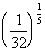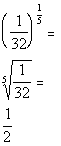*Rewrite exponent 1/5 as a fifth root

We are looking for the fifth root of 1/32 raised to the 1 power which is the same as just saying the fifth root of 1/32.

Our answer is 1/2 since the fifth root of 32 is 2.Answer/Discussion to 1b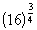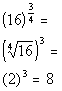*Rewrite exponent 3/4 as the fourth root being cubed

We are looking for the fourth root of 16 raised to the third power.

The fourth root of 16 is 2 and 2 cubed is 8.Answer/Discussion to 1c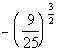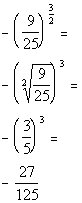*Rewrite exponent 3/2 as the square root being cubed

We are looking for the opposite of the square root of 9/25 raised to the third power.

The square root of 9/25 is 3/5 and 3/5 cubed is 27/125.  Taking the opposite we get -27/125.Answer/Discussion to 2a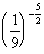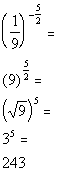We are looking for the square root of 9 raised to the fifth power.

The square root of 9 is 3 and 3 to the fifth power is 243.Answer/Discussion to 3a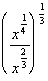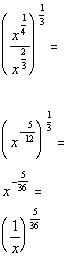Answer/Discussion to 3b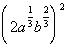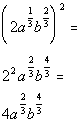Answer/Discussion to 4a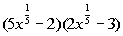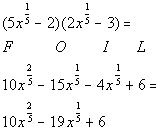Answer/Discussion to 5a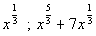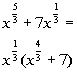*Factor out x to the 1/3Answer/Discussion to 6a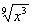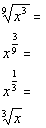*Simplify exponent